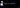# How implement merge sort algorithm in JavaScriptMerge sort algorithm was invented by John von Neumann in 1945. It is an efficient sorting algorithm using a divide and conquer approach.

• The Merge sort algorithm repeatedly divides the array into smaller chunks until we no longer divide the array into chunks.
• Then it repeatedly merges the chunks of the array to get a sorted array.

Example array : [3,4,2,1]

1. First, we need to divide the array into smaller chunks until we no longer divide the array into chunks.
``````        left       right
[3 , 4]     [2, 1]

       ``````
1. We need to merge the smaller chunks to get the sorted array.

Compare `3` and `4` they are already sorted so that we moved `2` and `1` where `2 > 1` so put `1` first and second `2`.

``````            left         right
[3 , 4]     [ 2, 1 ]

       

[3, 4]       [1 ,2]``````

Now, compare the left part index of `0` and right part index of `0` which are `3` and `1`.

Where `1` is smaller than `3` so that we removed `1` from the `right` part and push into the sorted list.

``````          left         right
[3 , 4]      [1, 2]

        

[3, 4]         

sorted array  [ 1 ]``````

Again we checked `3` and `2` and removed `2` from the right part and push into the sorted list

``````          left          right
[ 3 , 4 ]      [ 1, 2 ]

           

[3, 4]            [ ]

sorted array  [1,2]``````

Now, we need to merge the left part and right part with the sorted list.

``````          left         right
[3 , 4]       [1, 2]

         

[3, 4]         [ ]

sorted array  [ 1 ,2 ,3, 4]``````

## Algorithm implementation

Let’s implement a Merge sort algorithm in JavaScript.

``````function mergeSort(array,half = array.length/2){

if(array.length < 2){
return array  // it means we no longer divide the array
// into smaller chunks
}

const left = array.splice( 0,half ); //left part of  the array

return merger( mergeSort( left ),mergeSort( array ) )
}``````

In the above code, we are using a splice method to remove the left part of the array. The remaining part of the array is the right part.

Next, we need to implement a `merger` function which helps us to combine the `left` and `right` part of the array and returns the sorted list.

``````function merger(left, right) {
const arr = [  ];
while (left.length && right.length) {
if (left[ 0 ] < right[ 0 ]) {
arr.push( left.shift( ) ) // remove from the left part and push into
//the sorted array
} else {
arr.push( right.shift(  ) ) // remove from the right part and push into
//the sorted array
}
}
return [ ...arr, ...left, ...right ];
}``````

## Full code

``````function mergeSort(array,half = array.length/2){

if(array.length < 2){
return array
}

const left = array.splice(0,half); //left part of array

return merger(mergeSort(left),mergeSort(array))
}

function merger(left,right){

const arr = [];

while(left.length && right.length){
if(left < right ){
arr.push(left.shift())
}else{
arr.push(right.shift())
}
}

return [...arr,...left,...right];
}

console.log(mergeSort([10,5,3,8,2,6,4,7,9,1]));

//Output -> [1,2,3,4,5,6,7,8,9,10]``````## Codepen demo

Tested using mocha and chai

## Top Udemy Courses##### JavaScript - The Complete Guide 2020 (Beginner + Advanced)
45,614 students enrolled
52 hours of video content
View Course##### React - The Complete Guide (incl Hooks, React Router, Redux)
284,472 students enrolled
40 hours of video content
View Course##### Vue JS 2 - The Complete Guide (incl. Vue Router & Vuex)
130,921 students enrolled
21 hours of video content
View Course